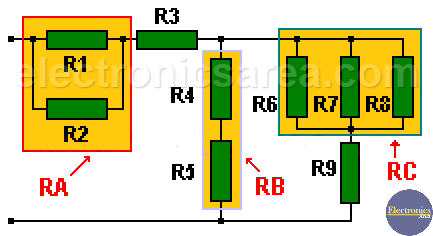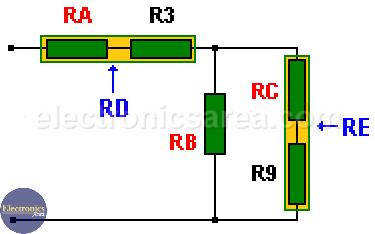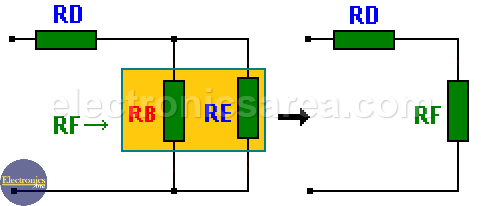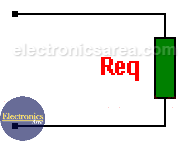# Series/Parallel Resistor Reduction

To make a Series or a Parallel Resistor Reduction, it is only necessary to do some simplifications using formulas we already know. The situation is different when you have to simplify a circuit that is composed of combinations of resistors in series and resistors in parallel.  In this case a Series/Parallel Resistor Reduction is needed.

## Series/Parallel resistor reduction procedure

To simplify a complex circuit and to obtain the equivalent resistance, we follow this procedure:

1 – You need to rearrange the circuit we want to simplify, to see clearly the component parts, and discover the parts that are already connected in series and parallel.

2 – We assign to each of these parts, a new name, such as RA, RB, RC, RD, etc. (look at the first image).

3 – We obtain the equivalent resistance of each part using the familiar formulas. (resistors in series and parallel resistances).

4 – We replace the parts in the original circuit with the values of the equivalent resistances (RA, RB, etc.) obtained in the previous step.

5 – We analyze the resulting circuit, and seek other possible combinations of resistance groups (parts) in series and parallel, which have been created.

6 – We repeat the process again from step 2 with different names for equivalent resistances to avoid confusion (e.g. RX, RY, RZ, etc.), until we obtain a single final equivalent resistance of the entire circuit.

Analyze the following image:Series/Parallel Resistor Reduction

Resistors values are:

R1 = 120, R2 = 250, R3 = 68, R4 = 47, R5 = 68. (all in Ohms).
R6 = 5, R7 = 4, R8 = 2, R9 = 1.2. (all in Kilo ohms).

First reduction

• RA = R1 and R2 in parallel,
RA = R1//R2 = R1 x R2 / (R1 + R2) = 120 x 250 / (120 + 250) = 81 ohms.
• RB = R4 and R5 in series,
RB = R4 + R5 = 47 + 68 = 115 ohms.
• RC = R6, R7 and R8 in parallel,
RC = R6//R7//R8 = 1/( 1/R6 + 1/R7 + 1/R8) = 1/( 1/ 5K + 1/4K + 1/2K) = 1053 ohms.

We replace the equivalent values, obtained in the previous steps, in the original circuit, and we get:Series/Parallel Resistor Reduction Procedure

We simplify the circuit again, and we get new equivalent resistances. then:

• RD = RA and R3 in series,
RD = RA + R3 = 81 + 68 = 149 ohms.
• RE = RC and R9 in series,
RE = RC + R9 = 1053 + 1200 = 2253 ohms.

And replacing these latest data, the following circuit is obtained:In the latter circuit it can be seen that RB and RE are in parallel, and we get a new RF equivalent resistance that is in series with RD:

RF = RB and RE in parallel,
RF = RB//RE = RB x RE / (RB + RE) = 115 x 2253 / (115 + 2253) = 109 Ohms.

RF is in series with RD. We add their values to obtain the final resistor value “Req”. That is the total equivalent resistance value of all the circuit.Then:

Req = RF and RD in series,
Req = RF + RD = 109 + 149 = 258 ohms.

•
•
• 13
•
•
•
•
•
13
Shares
•
13
Shares
•
•
• 13
•
•
•
•